# Test Prep Plan - Take a practice test

Take this practice test to check your existing knowledge of the course material. We'll review your answers and create a Test Prep Plan for you based on your results.
How Test Prep Plans work
1
2Based on your results, we'll create a customized Test Prep Plan just for you!
3Study smarter
Study more effectively: skip concepts you already know and focus on what you still need to learn.

# NMTA Math: Quadratic Equations Chapter Exam

Exam Instructions:

Choose your answers to the questions and click 'Next' to see the next set of questions. You can skip questions if you would like and come back to them later with the yellow "Go To First Skipped Question" button. When you have completed the practice exam, a green submit button will appear. Click it to see your results. Good luck!

### Page 1

#### Question 1 1. Find the roots of the equation.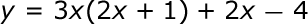#### Question 2 2. What is the b-value of the quadratic equation below?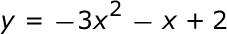#### Question 3 3. Solve the following equation.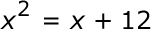#### Question 5 5. When completing the square for the quadratic equation shown below, how is c calculated?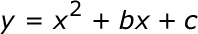### Page 2

#### Question 8 8. If the equation below told you a ball was 'h' feet in the air after 't' seconds, what would be the first step to finding out when it hit the ground?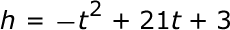#### Question 10 10. Which of the following are the solutions to the equation below?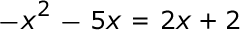### Page 3

#### Question 12 12. Rewrite the quadratic below in standard form: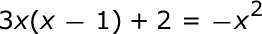### Page 4

#### Question 16 16. Find the solution to the equation below.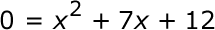#### Question 18 18. Find the solution to the equation below.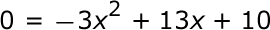### Page 5

#### Question 23 23. In order to complete the square on the following equation, what value would you add to both sides?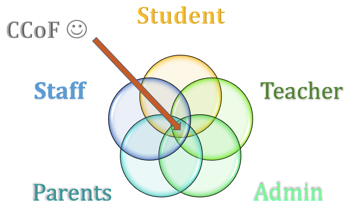#### Question 24 24. Use the quadratic formula to find the solutions to the equation below.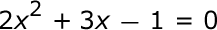#### Question 25 25. Find the roots of the equation below.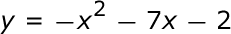#### NMTA Math: Quadratic Equations Chapter Exam Instructions

Choose your answers to the questions and click 'Next' to see the next set of questions. You can skip questions if you would like and come back to them later with the yellow "Go To First Skipped Question" button. When you have completed the practice exam, a green submit button will appear. Click it to see your results. Good luck!

Support